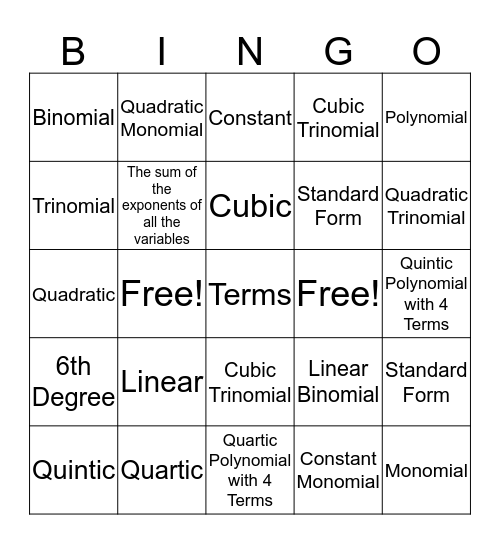# Classifying PolynomialsThis bingo card has a free space and 24 words: Linear, Quartic, Cubic, Quintic, Polynomial, Binomial, Trinomial, Quadratic Monomial, Free!, Cubic Trinomial, Standard Form, Quadratic, Linear Binomial, Standard Form, The sum of the exponents of all the variables, Constant Monomial, Quintic Polynomial with 4 Terms, Constant, Quadratic Trinomial, Free!, Monomial, 6th Degree, Cubic Trinomial and Quartic Polynomial with 4 Terms.

⚠ This card has duplicate items: Free! (2), Cubic Trinomial (2), Standard Form (2)

## Play Online